Multivariable Calculus : Parameterization & Surface Integrals

Example Questions

Example Question #1 : Parameterization & Surface Integrals

Evaluate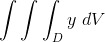, whereis the region below the plane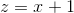, above the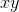plane and between the cylinders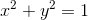, and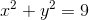.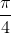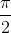Explanation:

We need to figure out our boundaries for our integral.

We need to convert everything into cylindrical coordinates. Remeber we are above theplane, this means we are above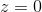.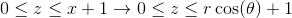The regionis between two circles, and.

This means that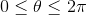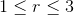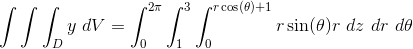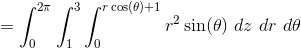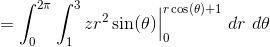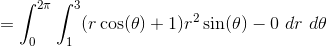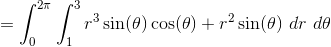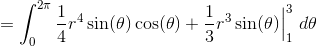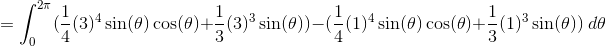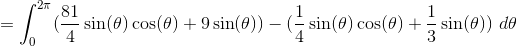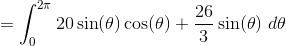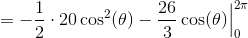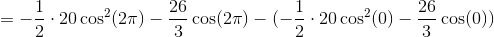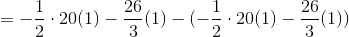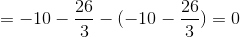Example Question #2 : Parameterization & Surface Integrals

Evaluate, whereis the region below the plane, above theplane and between the cylinders, and.Explanation:

We need to figure out our boundaries for our integral.

We need to convert everything into cylindrical coordinates. Remeber we are above theplane, this means we are above.The regionis between two circles, and.

This means that